## SMT Solving for Equalities and Uninterpreted Functions

Satisfiability Checking is the task of checking the existence of a satisfying solution for a logical formula. For propositional logic formulas, being Boolean combinations of propositions such as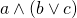, SAT solvers can be used to determine whether there is an assignment mapping to each proposition a value either true or false, such that substituting these values into the formula, the formula evaluates to true.

For the satisfiability check of quantifier-free first-order logic formulas over different theories, SAT solvers can be extended with theory solver modules, resulting in SAT-modulo-theories (SMT) solvers. Thereby we move from checking satisfiability for propositional logic formulas, such as, towards checking satisfiability for formulas such as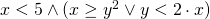. Such logics can be syntactically more rich as well as semantically more expressive than propositional logic, and allow the natural formalisation of a wide range of practical problems, e.g., in scheduling, planning, software verification, or controller synthesis.

This practical course aims at the design, implementation, and optimization of state-of-the-art decision procedures for SMT solving for the theories of equality logic and uninterpreted functions. Equality logic is an extension of propositional logic with a theory, allowing the usage of constants and variables having values from some domain, and equality as the only predicate. I.e., equality logic formulas are Boolean combinations of equalities between constants and variables. An example for a satisfiable equality logic formula is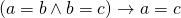, an example for an unsatisfiable formula is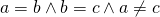. In the theory of uninterpreted functions, additionally to constants and variables, we can use function symbols in theory terms. The semantics of the function symbols is not specified (i.e. they are “uninterpreted”); the only constraint on their semantics is functional congruence, i.e.,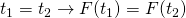for all theory terms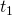,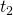and function symbols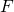. For example,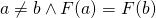is a satisfiable formula, whereas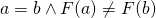is unsatisfiable.

The implementation will take place within the SMT-RAT project and can benefit from its manifold features. The resulting SMT solver will be tested on the official SMT-LIB benchmarks and be compared to state-of-the-art SMT solvers. Furthermore, we will form at least two teams and compare their solvers within a competition.

Slides

introduction

preliminaries

incremental_mis

improvements

Exercises